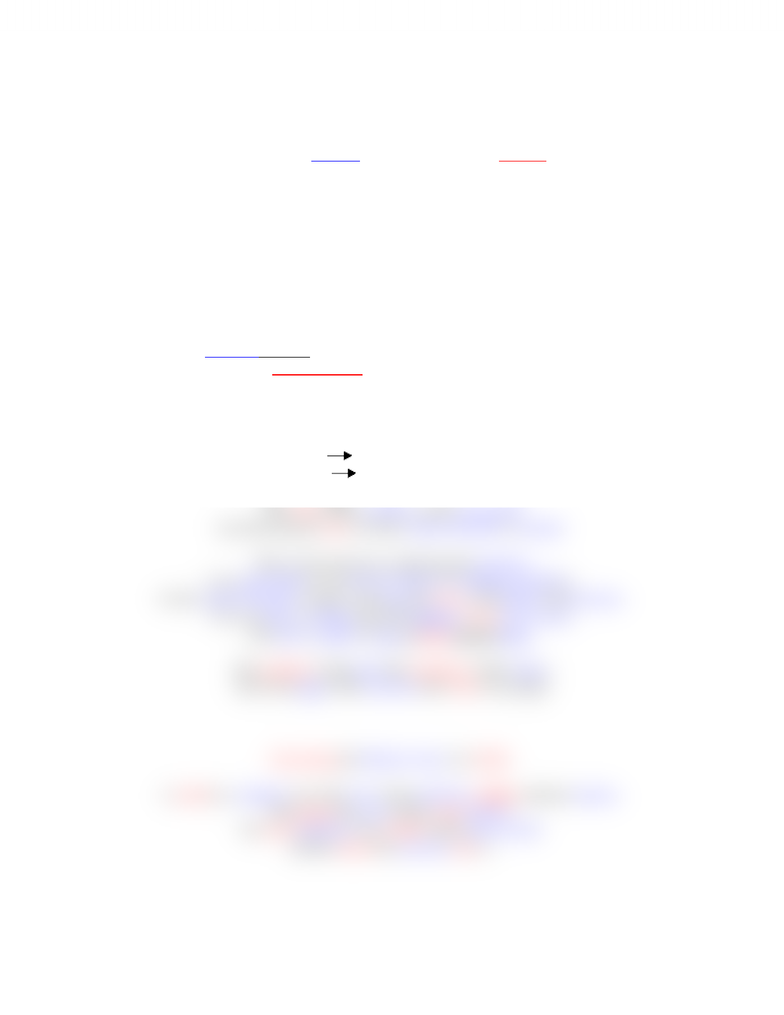# Chemistry 1027A/B Lecture Notes - Reaction Rate, Calcium Chloride

49 views2 pages
School
Department
ProfessorRates of Reaction
The rate of a reaction may be measured by following
the loss of a reactant, or the formation of a product.
Rate of reaction = amount of reactant used ÷ time.
Rate of reaction = amount of product formed ÷ time.
Three of the reactions which may be studied
to show how the rate can be changed are shown below.
They are
1) The reaction between calcium carbonate and dilute hydrochloric acid.
2) The reaction between sodium thiosulfate solution and hydrochloric acid.
3) The decomposition of hydrogen peroxide solution.
1) The reaction between calcium carbonate and dilute hydrochloric acid.
HCl + calcium carbonate calcium chloride + carbon dioxide + water.
HCl(aq) + CaCO3(s) CaCl2(aq) + CO2(g) + H2O(l)
The rate of this reaction can be measured
by following the rate at which carbon dioxide is formed.
This can be done by conducting the reaction
in an open flask on an electric balance (weighing machine).
As the carbon dioxide escapes to the air, the mass of the flask will decrease.
You can take a reading from the balance every 30 seconds,
then plot a graph of loss of mass against time.
The gradient of the plot (the steepness of the slope)
shows the rate of the reaction (how fast it is going)
Increasing the Surface Area of a Solid.
A solid in a solution can only react when particles collide with the surface.
The bigger the area of the solid surface,
the more particles can collide with it per second,
and the faster the reaction rate is.
Unlock document

This preview shows half of the first page of the document.
Unlock all 2 pages and 3 million more documents.

Already have an account? Log in

# Get access

Grade+
\$10 USD/m
Billed \$120 USD annually
Homework Help
Class Notes
Textbook Notes
40 Verified Answers
Study Guides
1 Booster Class
Class+
\$8 USD/m
Billed \$96 USD annually
Homework Help
Class Notes
Textbook Notes
30 Verified Answers
Study Guides
1 Booster Class